# Electric Field from Voltage

One of the values of calculating the scalar electric potential (voltage) is that the electric field can be calculated from it. The component of electric field in any direction is the negative of rate of change of the potential in that direction.

If the differential voltage change is calculated along a direction ds, then it is seen to be equal to the electric field component in that direction times the distance ds.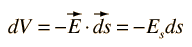The electric field can then be expressed as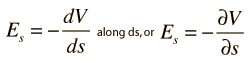This is called a partial derivative.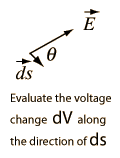For rectangular coordinates, the components of the electric field are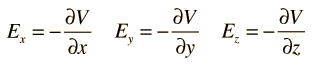Index

Voltage concepts

Electric field concepts

 HyperPhysics***** Electricity and Magnetism R Nave
Go Back

The expression of electric field in terms of voltage can be expressed in the vector form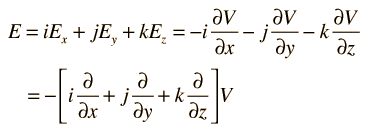This collection of partial derivatives is called the gradient, and is represented by the symbol ∇ . The electric field can then be written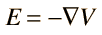Expressions of the gradient in other coordinate systems are often convenient for taking advantage of the symmetry of a given physical problem.

Index

Electric field concepts

Voltage concepts

 HyperPhysics***** Electricity and Magnetism R Nave
Go Back